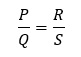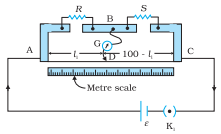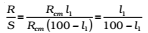It is the simplest practical application of the Wheatstone bridge that is used to measure an unknown resistance.

Principle: Its working is based on the principle of Wheatstone bridge.

When the bridge is balanced,Fig. A meter bridge. Wire AC is 1 m long. R is a resistance to be measured and S is a standard resistance.

### Construction:

It consists of a wire of length 1m and of uniform cross-sectional area stretched taut and clamped between two thick metallic strips bent at right angles, as shown.

The metallic strip has two gaps across which resistors can be connected. The endpoints where the wire is clamped are connected to a cell through a key.

One end of a galvanometer is connected to the metallic strip midway between the two gaps. The other end of the galvanometer is connected to a ‘jockey’.

The jockey is essentially a metallic rod whose one end has a knife-edge which can slide over the wire to make an electrical connection.

### Working:

The jockey can be moved along the wire. The portion AD of the wire has a resistance Rcml, where Rcm is the resistance of the wire per unit centimeter. The portion DC of the wire similarly has a resistance Rcm (100-l).

The four arms AB, BC, DA and CD [with resistances R, S, Rcml and Rcm(100-l)] obviously form a Wheatstone bridge with AC as the battery arm and BD the galvanometer arm. If the jockey is moved along the wire, then there will be one position where the galvanometer will show no current.Thus, once we have found out l1, the unknown resistance R is known in terms of the standard known resistance S byKnowing l and R, unknown resistance S can be determined.

Click on a star to rate it!

Average rating 4.5 / 5. Vote count: 26

No votes so far! Be the first to rate this post.

As you found this post useful...